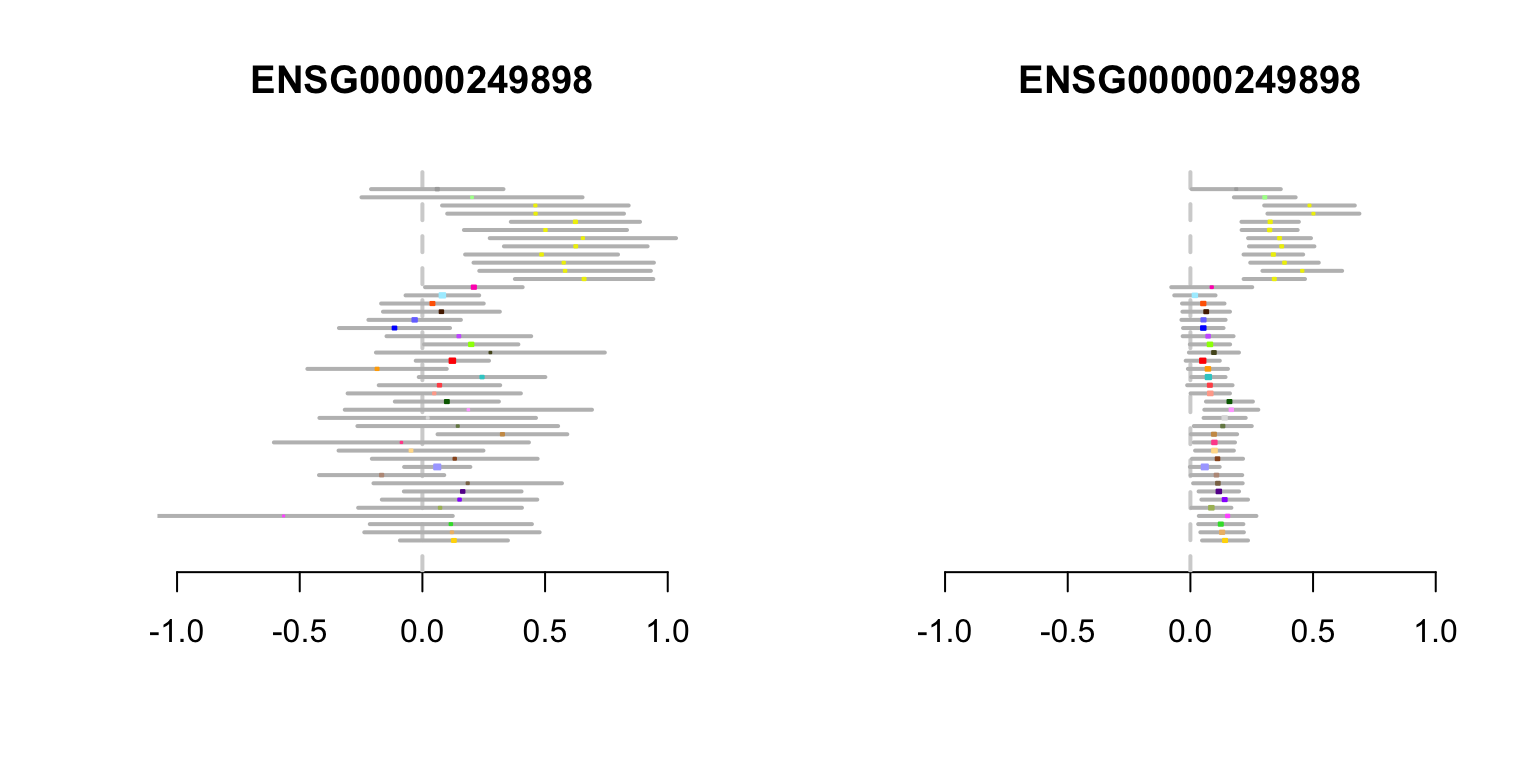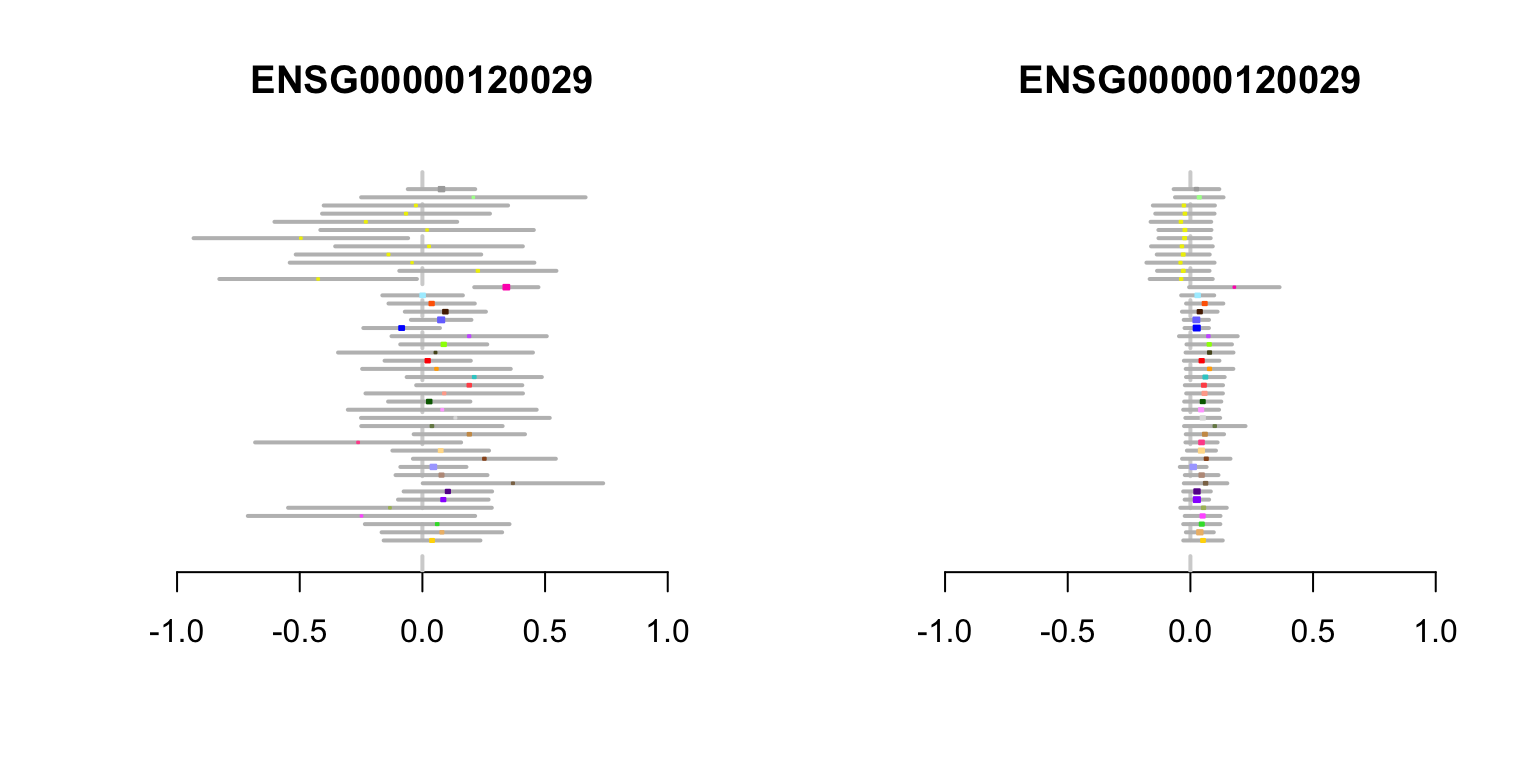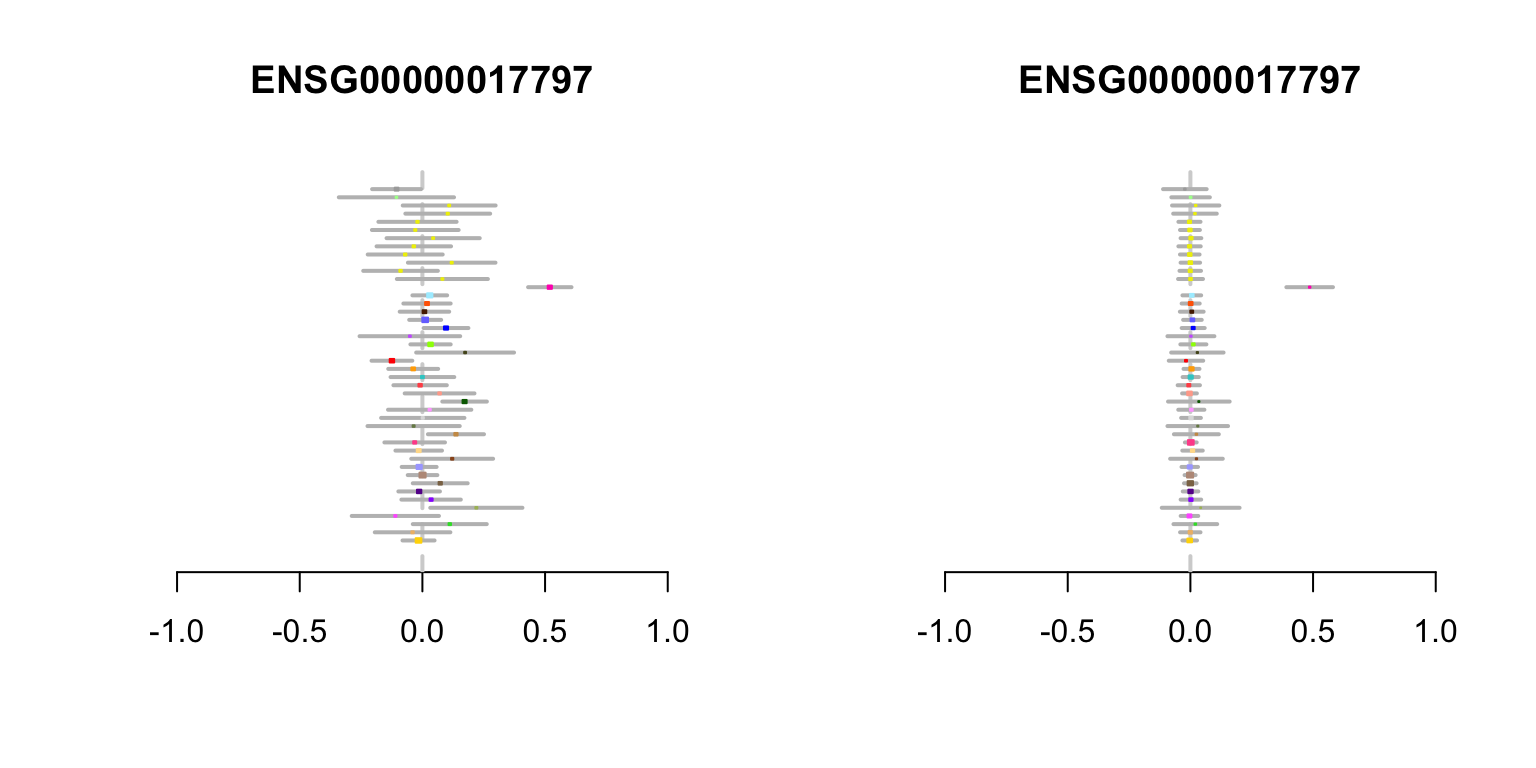In this notebook, we make the metaplots comparing the original effect estimates (effects of what?), and the posterior estimates obtained using the multivariate adaptive shrinkage model. What is the main result(s) we are showing in this notebook? What is the point of showing these plots. The plots below were used to create Fig. 4 of the paper.

First, we load the necessary packages into the R environment.

library(rmeta)

Please explain here what this next code chunk does. It looks like it is reading a bunch of data files. What data are being loaded?

source("../Scripts/normfuncs.R")

standard.error <- b.stat/z.stat

colnames(lfsr)  <- colnames(mar.var) <-
colnames(posterior.means) <-
colnames(z.stat)

posterior.betas <- standard.error * posterior.means
pm.beta.norm    <- het.norm(posterior.betas)

missing.tissues <- c(7,8,19,20,24,25,31,34,37)
uk3labels       <- read.table("../Data/uk3rowindices.txt")[,1]

In this next chunk you are defining a function. Can you briefly explain in 1-2 sentences what this function does, and how you will use it in the code chunks below?


# Can you please give a better name to this function than "newfunc.2"?
# Maybe a name like gen.metaplot?
newfunc.2 <- function (j) {
gtex.colors <- read.table('../Data/GTExColors.txt', sep = '\t',
comment.char = '')[-missing.tissues,2]
gtex.colors <- gtex.colors[uk3labels]

pm.beta.norm  <- pm.beta.norm[,uk3labels] # Shuffle columns.
z.shuffle     <- z.stat[,uk3labels]
b.shuffle     <- b.stat[,uk3labels]
post.var      <- mar.var[uk3labels]
post.bshuffle <- posterior.betas[,uk3labels]
sem.shuffle   <- standard.error[,uk3labels]
lfsr          <- lfsr[,uk3labels]
plot.title    <- strsplit(rownames(z.stat)[j], "[.]")[]

x <- as.numeric(post.bshuffle[j,])

layout(t(1:2))

metaplot(as.numeric(b.shuffle[j,]),as.numeric(sem.shuffle[j,]),xlab = "",
ylab = "",colors = meta.colors(box = as.character(gtex.colors)),
xlim = c(-1,1))
title(plot.title)

# Transform to posterior sd of beta.
sd <- as.numeric(sem.shuffle[j,]) * sqrt(as.numeric(post.var[j,]))
metaplot(x,sd,xlab = "",ylab = "",
colors = meta.colors(box = as.character(gtex.colors)),
xlim = c(-1,1))
title(plot.title)
}

## First example: MCPH1 gene

Our first example compares the MCPH1 original eQTL estimates against the posterior estimates.

What are the two plots showing? What is interesting about this example? What is the take-home message from this plot?

Please add labels to the horizontal and vertical axes. Which of these plots shows the original effect estimates, and which shows the posterior (“shrunk”) estimates?

three.ex.3 <-
which(rownames(z.stat) == "ENSG00000249898.3_8_6521432_T_C_b37")
newfunc.2(three.ex.3)# This line of code doesn't seem to do anything. Why is it included?
whole.blood.spec <-
which(rowSums(pm.beta.norm[,-44]<0.5)==43&(rowSums(lfsr[,1:44]<0.05)>=40))

## Second example: FLJ13114 gene

five.ex <- which(rownames(z.stat) == "ENSG00000120029.8_10_103924251_G_A_b37")

# This line of code doesn't seem to do anything. Why is it included?
testes.spec <-  which(rowSums(pm.beta.norm[,-40]<0.5) == 43 &
(rowSums(lfsr[,1:44]<0.05)>=40))[1:10]

newfunc.2(five.ex)## Third example: RALBP1 gene

wholebloodfour <-
(which(rownames(z.stat) == "ENSG00000017797.7_18_9488704_C_T_b37"))
newfunc.2(wholebloodfour)## Session Information

print(sessionInfo())
# R version 3.4.0 (2017-04-21)
# Platform: x86_64-apple-darwin15.6.0 (64-bit)
# Running under: OS X El Capitan 10.11.6
#
# Matrix products: default
# BLAS: /Library/Frameworks/R.framework/Versions/3.4/Resources/lib/libRblas.0.dylib
# LAPACK: /Library/Frameworks/R.framework/Versions/3.4/Resources/lib/libRlapack.dylib
#
# locale:
#  en_US.UTF-8/en_US.UTF-8/en_US.UTF-8/C/en_US.UTF-8/en_US.UTF-8
#
# attached base packages:
#  grid      stats     graphics  grDevices utils     datasets  methods
#  base
#
# other attached packages:
#  rmeta_2.16
#
# loaded via a namespace (and not attached):
#   compiler_3.4.0  backports_1.0.5 magrittr_1.5    rprojroot_1.2
#   tools_3.4.0     htmltools_0.3.6 yaml_2.1.14     Rcpp_0.12.10
#   stringi_1.1.5   rmarkdown_1.5   knitr_1.15.1    stringr_1.2.0
#  digest_0.6.12   evaluate_0.10